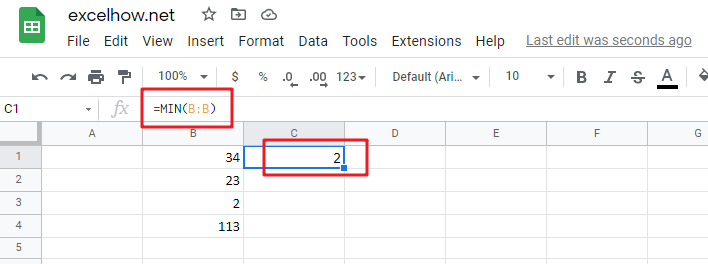# Google Sheets MIN Function

This post will guide you how to use Google Sheets MIN function with syntax and examples in Google Spreadsheet.

## Description

The Google Sheets MIN function returns the smallest numeric value from the numbers that you provided. Or returns the smallest value in the array.

The MIN function is a build-in function in Google Sheets and it is categorized as a Statistical Function.

## Syntax

The syntax of the MIN function is as below:

= MIN(num1,[num2,…numn])

Where the MIN function arguments are:

• num1 -This is a required argument. A number, or a reference to a number or a range that contains numeric values
• Num2,…Numn – This is an optional argument.  A number, or a reference to a number or a range that contains numeric values

Note:

• The MIN function will not compare empty cells
• The MIN function can accept up to 255 arguments with numeric value.
• The MIN function will ignore Boolean values (TRUE and FALSE)
• The MIN function will ignore numbers that the format is text.
• The returned value of The MIN function is a numeric value.

## Google Sheets MIN Function Examples

The below examples will show you how to use Google Sheets MIN Function to get the smallest value form the numbers.

#1 To get the smallest value from the column A, just using the following Google Sheets formula:

`=MIN(B:B)`Calculate The Average Of The Last 3, 5, Or N Numeric Values In Google Sheets

Related Posts

Cap percentage values between 0 and 100

This article will talk about how to cap the percentage values between 0% and 100% in Microsoft Excel Spreadsheet or Google Sheets. If you are a newbie on Excel or google Sheets, you may be able to do this by ...

Calculate Cap Percentages to Specific Value

This article will talk about how to limit the cap percentage of a given amount to a given value in Microsoft Excel Spreadsheet or Google Sheets. If you are a newbie on Excel or google Sheets, you may be able ...

Find the Closest Data to the Data Provided in Google Sheets

In our daily work, we may encounter such an issue that to find the closest value to a certain value. In fact, google sheets internal functions can help us solve this problem. In today’s article, we will show you how ...

Basic Array Formula With Examples in Google Sheets

Are you searching for an article for getting the basic array formula with different examples for better understanding? Then congratulations because you have just landed on the right article. In this article, you would get to know the basic array ...

Calculate Average Of Last 5 Or N Values In Columns in Google Sheets

Suppose you come across a task where you need to calculate the average of the last 2 or 3 numeric values in google sheets, then what would you do? If you are new to google sheets, then your first attempt ...

Sidebar